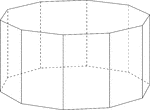Decagonal Prism

Illustration of a decagonal prism with regular decagons for bases and rectangular faces. The hidden…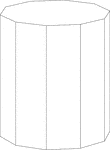Decagonal Prism

Illustration of a right decagonal prism with regular decagons for bases and rectangular faces.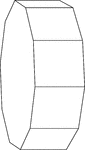Decagonal Prism Resting On Its Side

Illustration of a right decagonal prism with regular decagons for bases and rectangular faces. The prism…Swimming Pool Shaped Like A Decagonal Prism

Illustration of a swimming pool and water hose that is in the shape of a hollow regular decagonal prism…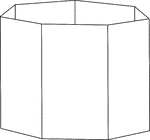Heptagonal/Septagonal Prism

Illustration of a hollow right heptagonal/septagonal prism with regular heptagons/septagons for bases…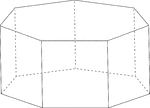Heptagonal/Septagonal Prism

Illustration of a regular right heptagonal/septagonal prism with regular heptagons/septagons for bases…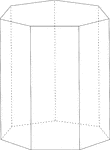Heptagonal/Septagonal Prism

Illustration of a right heptagonal/septagonal prism with regular heptagons/septagons for bases and rectangular…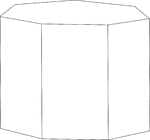Heptagonal/Septagonal Prism

Illustration of a right heptagonal/septagonal prism with regular heptagons/septagons for bases and rectangular…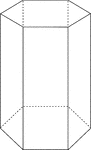Hexagonal Prism

Illustration of a right hexagonal prism with hidden edges shown.Hexagonal Prism

Illustration of a right hexagonal prism with a height less than the length of the edge of the hexagon.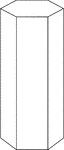Hexagonal Prism

Illustration of a right hexagonal prism with a height greater than the length of the edge of the hexagon.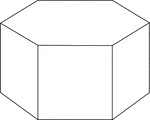Hexagonal Prism

Illustration of a right hexagonal prism with hexagons for bases and square faces.Hexagonal Prism

Illustration of a right hexagonal prism with hexagons for bases and square faces. The hidden edges are…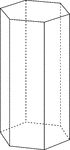Hexagonal Prism

Illustration of a right hexagonal prism with hexagons for bases and rectangular faces. The height of…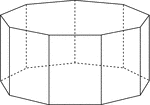Nonagonal Prism

Illustration of a right nonagonal prism with regular nonagons for bases and rectangular faces. The hidden…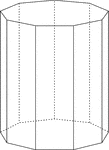Nonagonal Prism

Illustration of a right nonagonal prism with regular nonagons for bases and rectangular faces. The height…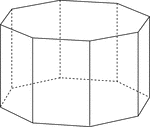Octagonal Prism

Illustration of a right octagonal prism with regular octagons for bases and rectangular faces. The hidden…Octagonal Prism

Illustration of a right octagonal prism with regular octagons for bases and rectangular faces. The height…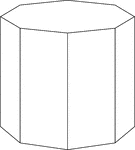Octagonal Prism

Illustration of a right octagonal prism with octagons for bases and rectangular faces. The height of…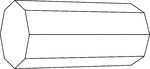Octagonal Prism Resting On Side

Illustration of a right octagonal prism, with octagons for bases and rectangular faces, that is resting…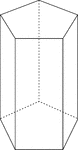Pentagonal Prism

Illustration of a right pentagonal prism with regular pentagons for bases and rectangular faces. The…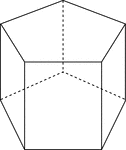Pentagonal Prism

Illustration of a right pentagonal prism with regular pentagons for bases and rectangular faces. The…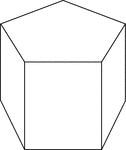Pentagonal Prism

Illustration of a right pentagonal prism with regular pentagons for bases and rectangular faces. The…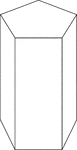Pentagonal Prism

Illustration of a right pentagonal prism with regular pentagons for bases and rectangular faces. The…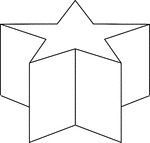Star-Shaped Decagonal Prism

Illustration of a non-regular decagonal prism in the shape of a star. Then ends/bases are made of star-shaped…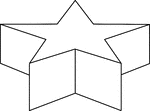Star-Shaped Decagonal Prism

Illustration of a non-regular decagonal prism in the shape of a star. Then ends/bases are made of star-shaped…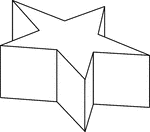Star-Shaped Decagonal Prism

Side view of a non-regular decagonal prism in the shape of a star. Then ends/bases are made of star-shaped…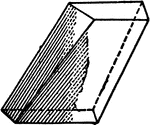An illustration of a prismatoid with faces that are quadrilaterals.2 Octagonal Prisms

Illustration of 2 right octagonal prisms with congruent bases, but different heights. The height of…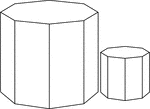2 Similar Octagonal Prisms

Illustration of 2 Similar right octagonal prisms. The height and length of the edges of the smaller…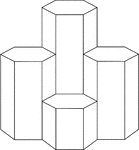4 Hexagonal Prisms

A cluster of 4 right hexagonal prisms with congruent bases, but varying heights.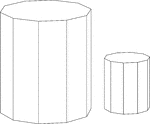Similar Decagonal Prisms

Illustration of 2 similar right decagonal prisms. Both have regular decagons for bases and rectangular…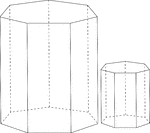Similar Heptagonal/Septagonal Prisms

Illustration of 2 similar right heptagonal/septagonal prisms. Both have regular heptagons/septagons…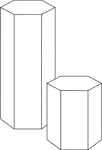Similar Hexagonal Prisms

Illustration of 2 similar right hexagonal prisms. The height of the prism and length of the side of…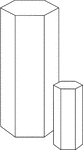Similar Hexagonal Prisms

Illustration of 2 similar right hexagonal prisms. The height of the prism and length of the side of…